Introduction to Sequential Logic; Latches

Tom Kelliher, CS 240

Apr. 2, 2010

Announcements

First Xilinx assignment; re-scheduled exam.

From Last Time

Finished Xilinx introduction lab.

Outline

1. Sequential logic.

2. SR latch.

3. D latch.

Flip-flops

Sequential Logic

1. Combinational logic is nice but...

2. Sequential logic: introduces notion of memory.

3. Synchronous vs. asynchronous circuits.

There will always be some asynchronous elements in a circuit which interfaces to the real world environment.

4. Clock: frequency, period, edges, duty-cycle.

Non-overlapping clocks.

5. How can we achieve memory?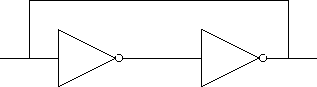This is the basic idea, to be modified for actual use.

6. General model of a sequential circuit: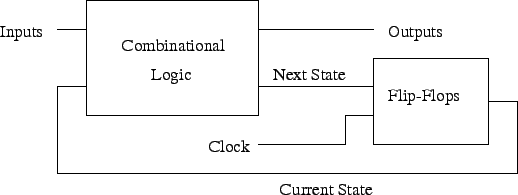SR Latch

1. Active low inputs.

2. Schematic: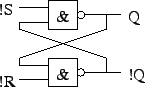3. Operation: three valid, one invalid input.

4. Asynchronous.

Clocked SR Latch

1. Schematic: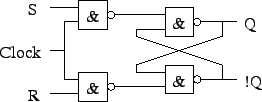Behavior.

2. Transparent when clock is high.

Latched when clock is low.

3. Problem with use in circuits: double clocking.

A solution: non-overlapping clocks. (Achieved with master-slave flip-flops.)

D Latch

1. SR latches inconvenient when storing data from, say, an ALU.

2. D latch stores data directly: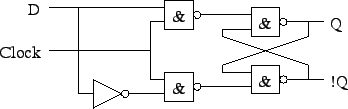(Think of this as a logic primitive.)

Thomas P. Kelliher 2010-04-01
Tom Kelliher